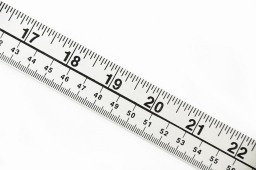# Calculate 63894

In the 8A class, the average height of 12 girls is 156 cm, and the average height of 14 boys is 172 cm. Calculate the average number of students in 8A.

x =  164.6154 cm

### Step-by-step explanation:Did you find an error or inaccuracy? Feel free to write us. Thank you!

Tips for related online calculators
Looking for help with calculating arithmetic mean?
Looking for a statistical calculator?
Do you want to convert length units?

#### Grade of the word problem:

We encourage you to watch this tutorial video on this math problem: